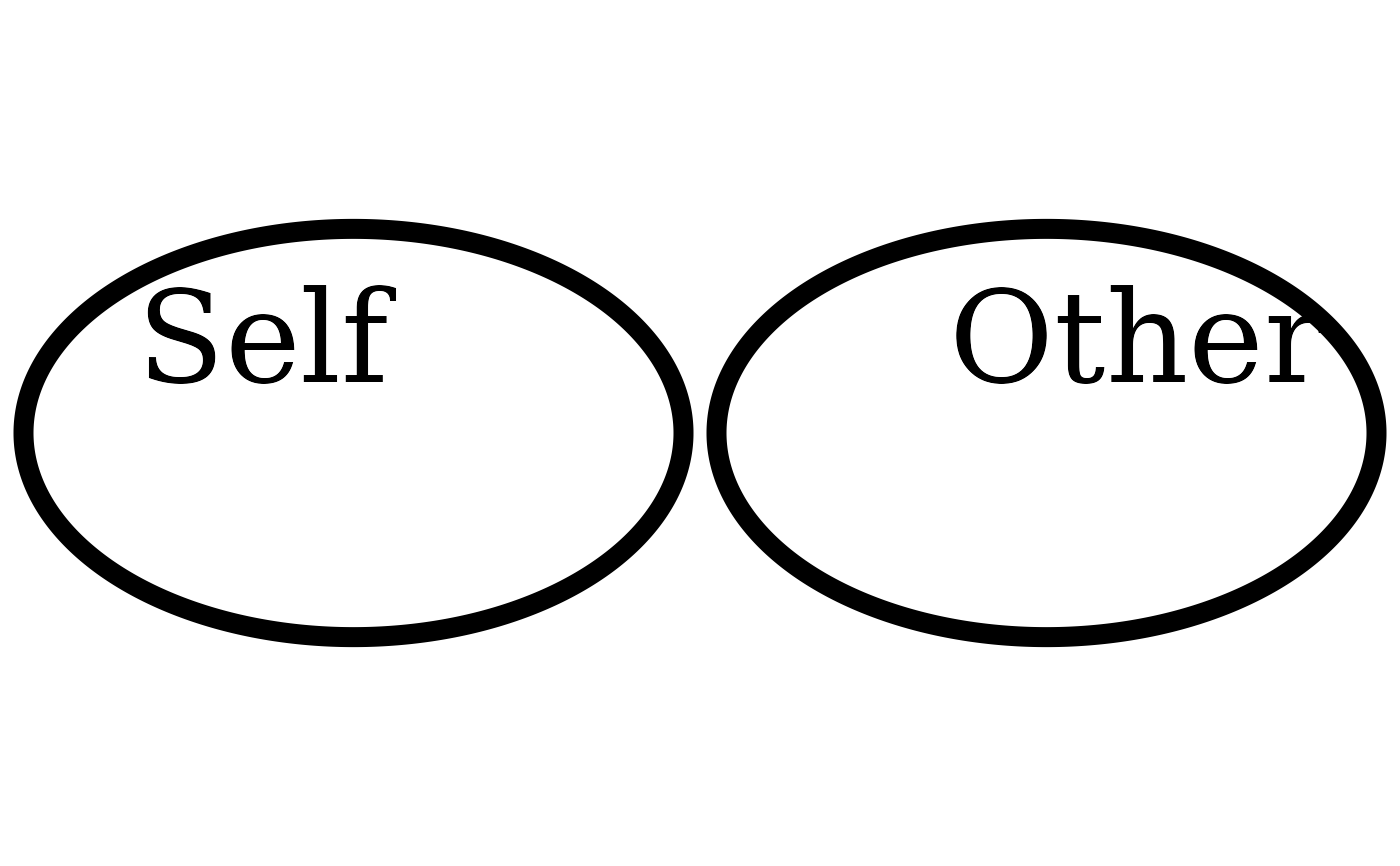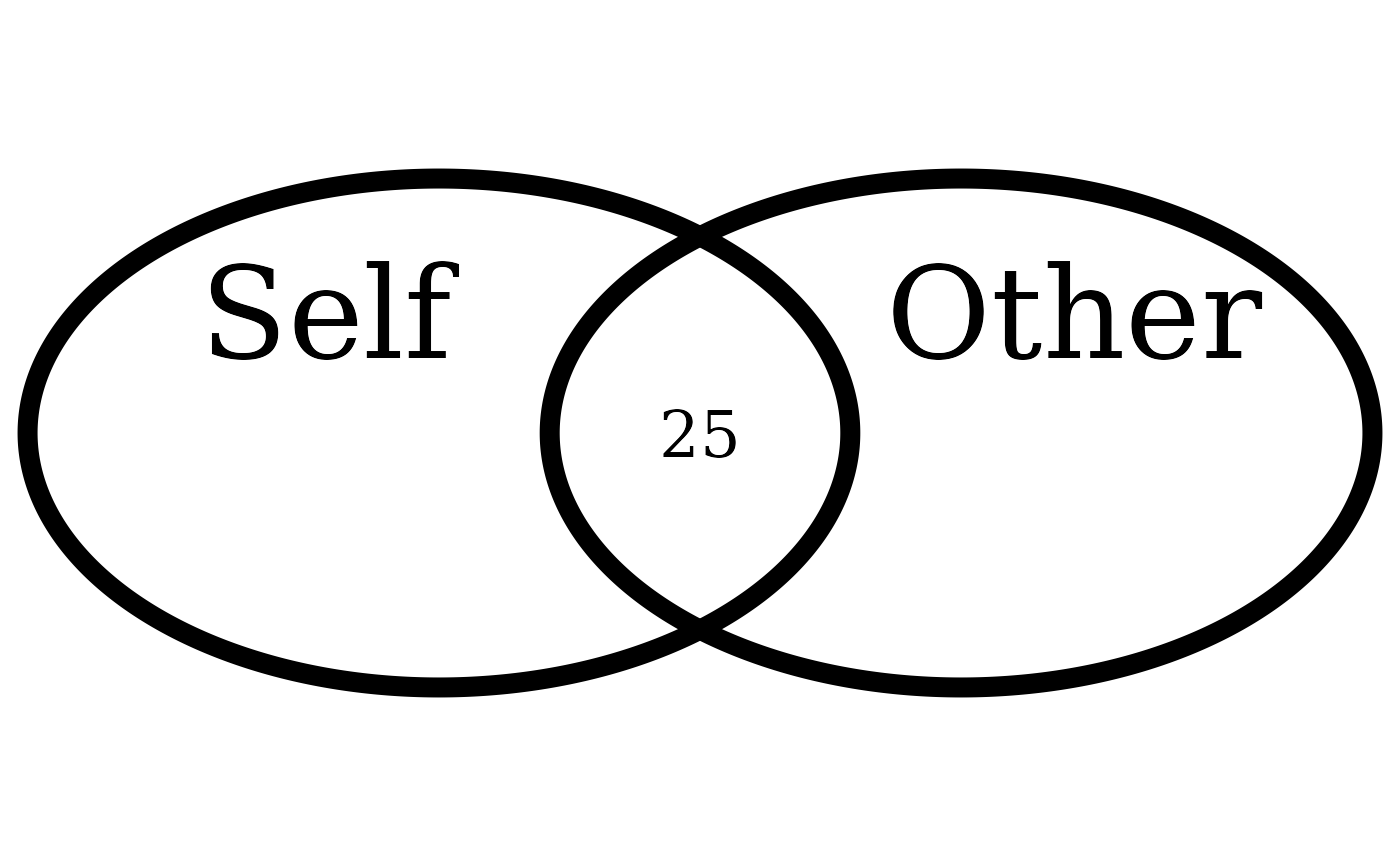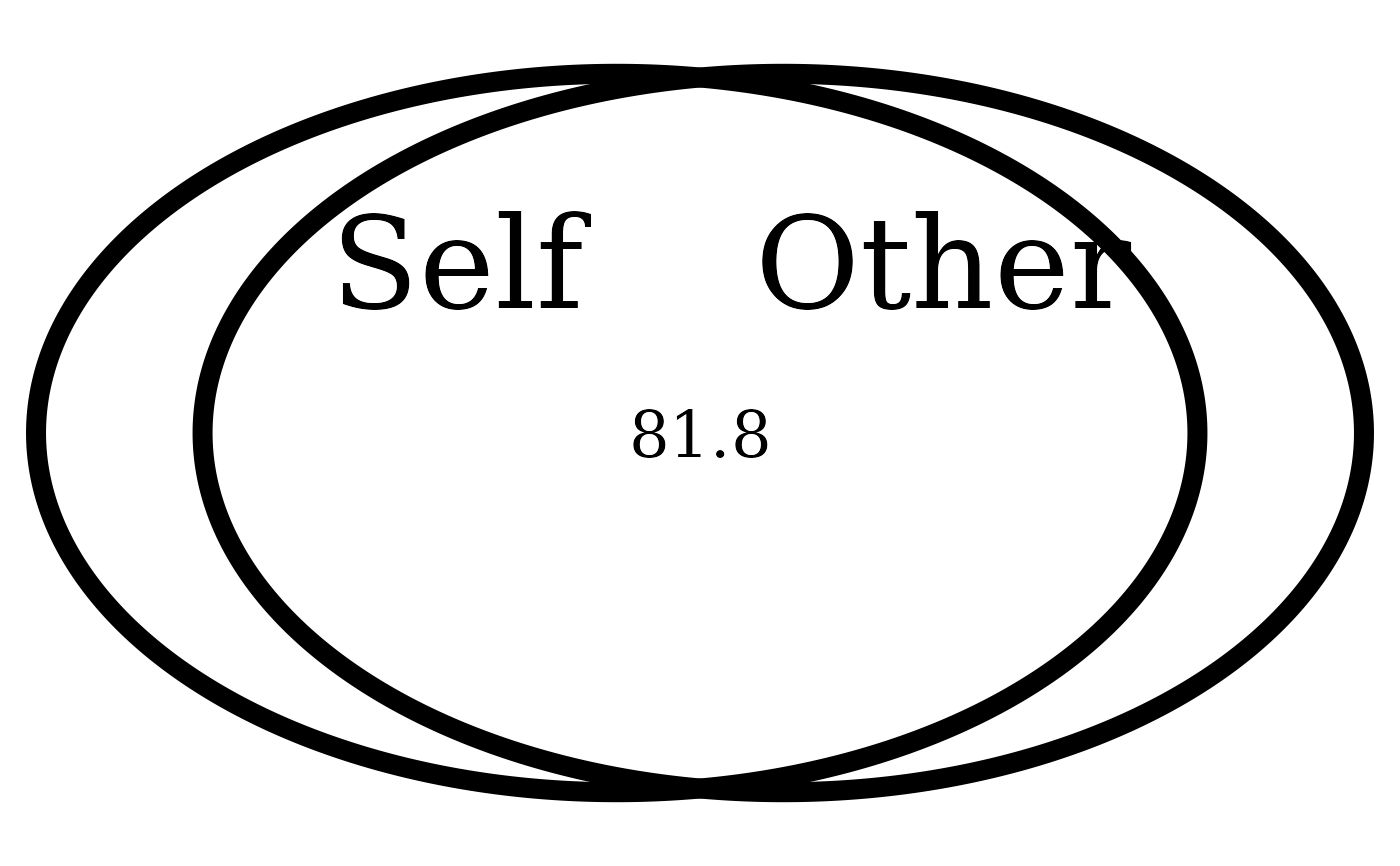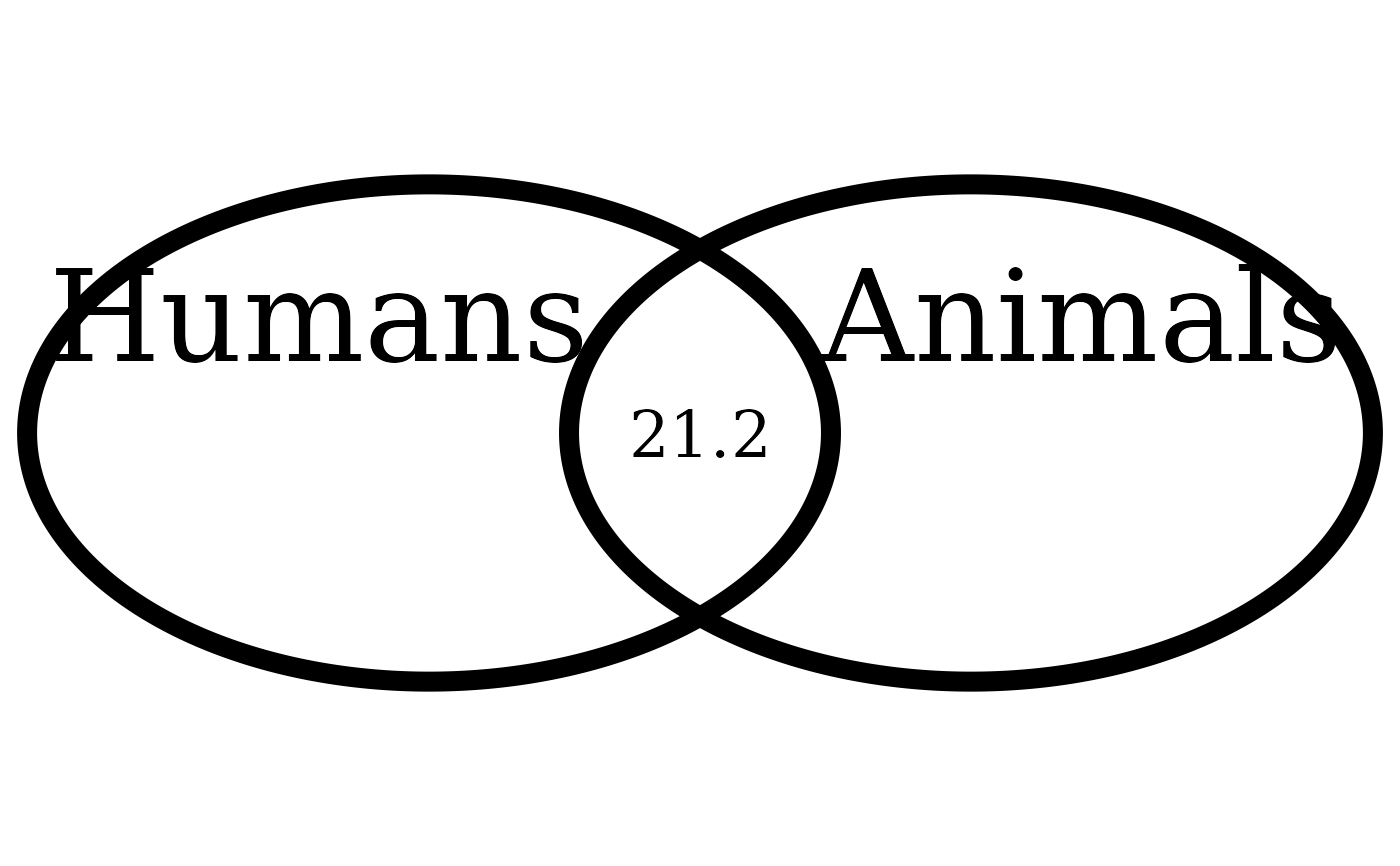Interpolating the Inclusion of the Other in the Self Scale (IOS; self-other merging) easily. The user provides the IOS score, from 1 to 7, and the function will provide a percentage of actual area overlap between the two circles (i.e., not linear overlap), so it is possible to say, e.g., that experimental group 1 had an average overlap of X% with the other person, whereas experimental group 2 had an average overlap of X% with the other person.

## Usage

overlap_circle(response, categories = c("Self", "Other"), scoring = "IOS")

## Arguments

response

The variable to plot: requires IOS scores ranging from 1 to 7 (when scoring = "IOS").

categories

The desired category names of the two overlapping circles for display on the plot.

scoring

One of c("IOS", "percentage", "direct"). If scoring = "IOS", response needs to be a value between 1 to 7. If set to "percentage" or "direct", responses need to be between 0 and 100. If set to "direct", must provide exactly three values that represent the area from the first circle, the middle overlapping area, and area from the second circle.

## Value

A plot of class gList, displaying overlapping circles relative to the selected score.

## Details

The circles are generated through the VennDiagram::draw.pairwise.venn() function and the desired percentage overlap is passed to its cross.area argument ("The size of the intersection between the sets"). The percentage overlap values are interpolated from this reference grid: Score of 1 = 0%, 2 = 10%, 3 = 20%, 4 = 30%, 5 = 55%, 6 = 65%, 7 = 85%.

For a javascript web plugin of a continuous version of the Inclusion of Other in the Self (IOS) task (instead of the pen and paper version), for experiments during data collection, rather than data analysis, please see: https://github.com/jspsych/jspsych-contrib/tree/main/packages/plugin-ios

## Examples

# Score of 1 (0% overlap)
overlap_circle(1)# Score of 3.5 (25% overlap)
overlap_circle(3.5)# Score of 6.84 (81.8% overlap)
overlap_circle(6.84)# Changing labels
overlap_circle(3.12, categories = c("Humans", "Animals"))# \donttest{
# Saving to file (PDF or PNG)
plot <- overlap_circle(3.5)ggplot2::ggsave(plot,
file = tempfile(fileext = ".pdf"), width = 7,
height = 7, unit = "in", dpi = 300
)
# Change for your own desired path
# }